### Home > A2C > Chapter Ch11 > Lesson 11.2.5 > Problem11-104

11-104.
1. Without graphing, find where each of the following curves crosses the x-axis. (Find the exact points!) Homework Help ✎

1. f (x) = x2x − 12

2. f (x) = 2x2 − 3x − 9

3. f (x) = 2x2 + x − 7

4. f (x) = 3x2 − 2x + 7

5. f (x) = 3x3 + 2x2 − 8x

6. f (x) = 2x3 + 2x2 + 13x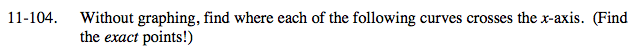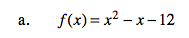Factor.
f(x) = (x + 3)(x − 4)

(−3, 0) and (4, 0)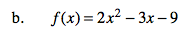Use the quadratic formula.

(−1.5, 0) and (3, 0)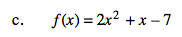See part (b).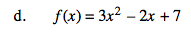See part (b).

It does not intersect the x-axis.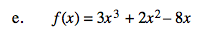x is a common factor.

After you factor out x, factor or use the quadratic formula to find the roots of the rest.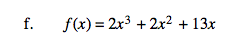See part (e).